# Rice’s Theorem

Every non-trivial property,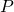, of the language of Turing Machines is undecidable. This is Rice’s Theorem.

Becauseis non-trivial there is some Turing Machine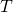such that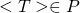and some other Turing Machine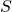such that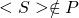.

## Proofis undecidable. This can be proved using contradiction.

Suppose to the contrary that that there exists a TM,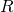, that decides. This machine takes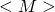as an input. It accepts if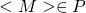and rejects otherwise.

Using, build another TM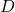as follows

On input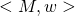1. Construct a new TM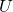as follows
1. On input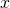, Run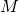on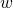.
2. Ifaccepts, runon.
3. Ifaccepts, accept.
2. Runon. Ifaccepts(implying thathas property), accept. Else, reject.

The machinetakesas an input. It accepts ifsatisfies the property. This implies thatandshare the same language. This will only hold true ifaccepts. In essence, this is a machine that decides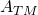. We say thatreduces to.

Sinceis undecidable, the propertyis also undecidable.

## Example

Using Rice’s theorem makes the problem of proving the undecidability of languages pretty straightforward. You have to identify the property and prove that it is non-trivial. Consider the following problem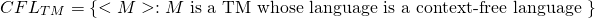The propertyin this case is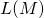is context-free.is indeed a property of the language of TMs because for any 2 machinesand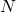such that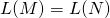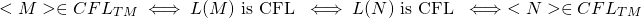is non-trivial because there is at least one TM that belongs to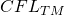(a TM that accepts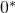) and at least one TM that doesn’t belong to(a TM that accepts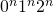).

By Rice’s Theorem,is undecidable.